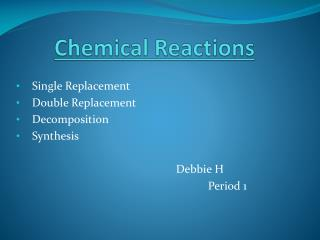DownloadDownload PresentationChemical Reactions

# Chemical Reactions

Télécharger la présentation## Chemical Reactions

- - - - - - - - - - - - - - - - - - - - - - - - - - - E N D - - - - - - - - - - - - - - - - - - - - - - - - - - -
##### Presentation Transcript

1. Chemical Reactions Single Replacement Double Replacement Decomposition Synthesis Debbie H Period 1

2. Single Replacement Magnesium and Hydrochloric Acid equals Magnesium Chloride and Hydrogen Mg(s) + HCl(Aq)

3. Single Replacement Magnesium and Hydrochloric Acid equals Magnesium Chloride and Hydrogen Mg(s) + HCl(Aq) Balanced: Mg(s) + 2HCl(Aq) MgCl2(Aq) + H2(g) Use table J to figure out if these reactions will happen.

4. Double Replacement Copper (II) nitrate and sodium hydroxide equals cobalt (II) hydroxide and sodium nitrate Co(NO3)2 (Aq) + NaOH(Aq)

5. Double Replacement Copper (II) nitrate and sodium hydroxide equals cobalt (II) hydroxide and sodium nitrate Co(NO3)2 (Aq) + NaOH(Aq) Balanced: Co(NO3)2(Aq) + 2NaOH(Aq) Co(OH)2(S) + 2NaNO3(Aq) Two aqueous solutions form a solid and a different aqueous solution.

6. Decomposition Oxygen difluoride decomposes into oxygen and fluorine. OF2(g)

7. Decomposition Oxygen difluoride decomposes into oxygen and fluorine. OF2(g) Balanced: 2OF2(g)O2(g) + 2F2 (g) The same number of atoms exists at the start of a chemical reaction as at the end, but they bond together differently.

8. Synthesis Calcium reacts with nitrogen to form calcium nitride Ca(s)+ N2(g)

9. Synthesis Calcium reacts with nitrogen to form calcium nitride Ca(s) + N2(g) Balanced: 3Ca(s) + N2(g)Ca3N2(S) Two separate elements form into one larger chemical substance.

10. Summary: Using Table E and Table J we are able to figure out the order of the equations. Using the reference table we can figure out the amount of ions that are in each element. After, balance the equation into the smallest possible ratio.